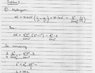# Electron in a box, size of the box

• warfreak131

## Homework Statement

I have a test in my physics class on tuesday, and as a study guide, our professor gave us a sample test and the solutions. I worked through most of it, except for this one question. I don't understand his method to solving it, I was hoping you guys had a solution. I have already email him about it, but he has yet to answer.

When an electron decays from the n=2 state to the n=1 state, it emits a photon. Determine the size of the box, L, for which the energy of this photon equals the energy of the analogous n=2 to n=1 transition in hydrogen.

## The Attempt at a Solution

HIS attempt at the solution is in the attached jpeg.

I understand the process of finding the energy of the photon, that's very easy, but in finding the size of the box, he introduces this pi2 term, and instead of doing the 1/n2 - 1/m2, he simply does n2-m2

#### Attachments

•Solution.jpg
54.7 KB · Views: 428

Are you familiar with the infinite square well? That's the box your professor is talking about.

I understand the process of finding the energy of the photon, that's very easy, but in finding the size of the box, he introduces this pi2 term, and instead of doing the 1/n2 - 1/m2, he simply does n2-m2

Your professor is considering the energy levels for a particle in a box. look here

Code:
[PLAIN]http://en.wikipedia.org/wiki/Particle_in_a_box
[/PLAIN] [Broken]

For one dimensional box of length L, the energy levels are

$$E_n=\frac{n^2\hbar^2 \pi^2}{2mL^2}$$

so

$$\Delta E=E_2-E_1=\frac{\hbar^2 \pi^2}{2mL^2}(2^2-1^2)$$

Last edited by a moderator:
Are you familiar with the infinite square well? That's the box your professor is talking about.

Yes, but I'm still not sure what he's doing.

Your professor is considering the energy levels for a particle in a box. look here

Code:
[PLAIN]http://en.wikipedia.org/wiki/Particle_in_a_box
[/PLAIN] [Broken]

For one dimensional box of length L, the energy levels are

$$E_n=\frac{n^2\hbar^2 \pi^2}{2mL^2}$$

so

$$\Delta E=E_2-E_1=\frac{\hbar^2 \pi^2}{2mL^2}(2^2-1^2)$$

ooooo, i see.

thanks!

Last edited by a moderator: